Courses

# Laws Of Crystallography - Solid State, Physical Chemistry | EduRev Notes

## Physical Chemistry

Created by: Asf Institute

## Chemistry : Laws Of Crystallography - Solid State, Physical Chemistry | EduRev Notes

The document Laws Of Crystallography - Solid State, Physical Chemistry | EduRev Notes is a part of the Chemistry Course Physical Chemistry.
All you need of Chemistry at this link: Chemistry

Laws of Crystallography

The geometric crystallography is based on the three fundamental laws:

(i) The laws of constancy of interfacial angles: The crystals of a substance can have different shapes depending upon the number and size of the faces but the angle at which the two adjacent faces intersect remains always constant.

(ii) Hauy’s law of rationality of indices: The intercepts of any face of plane of a crystal on suitable crystallographic axes can be expressed by small multiples of three unit distances a, b, c or some simple integral multiple (m, n, p) of these unit distances, i.e., ma: nb: pc or fraction of whole numbers.

(iii) The law of constancy of symmetry: according to this law, all crystals of the same substance possess the same elements of symmetry. A crystal possesses three types of symmetry:

(a) Plane of symmetry: A crystal is supposed to have a plane of symmetry when an imaginary plane passing through the centre of a crystal can divide it into two parts which are mirror images to each other. The standard rational for a plane of symmetry is represented by σ.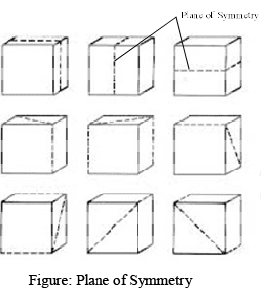(b) Axis of Symmetry: An axis of symmetry represents a line about which the crystal may be rotated so that is represents the same appearance more than one during the complete revolution.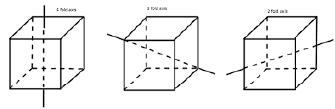(c) Centre of Symmetry: A crystal is supposed to have centre of symmetry if every face has an identical face at an equal distance on the opposite side of this corner.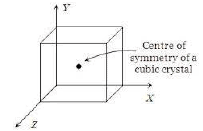The total number of planes, axis and centre of symmetries possessed by a crystal are called as elements of symmetry, e.g., a cubic crystal (say NaCl) possesses in all 23 elements of symmetry. There are three rectangular planes of symmetry, six diagonal planes of symmetry, three four folds axes of symmetry at right angles to each other, four three folds axes of symmetry and six two fold axes of symmetry along wit h one centre of symmetry lying at the centre of cube.

In a cubic crystal

Planes of symmetry = 3 + 6 = 9
Axes of symmetry = 3 + 4 + 6 = 13
Centre of symmetry = 1
Total elements of symmetry = 9 + 13 + 1 = 23

Terms and Concepts

(i) Space lattice or crystal lattice : It may be defined as a regular three dimensional arrangement of identical points in space.
The positions which are occupied by atoms, ions or molecules in the crystal lattice are called lattice points or lattice sites.

(ii) Unit Cell : A space lattice can be sub-divided into a number of small cells known as unit cells. It can be defined as the smallest block from which entire crystal can be built up by its translational repetition in three dimensions.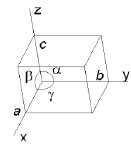Unit cell

The unit cell shown in figure is characterizes by a, b & c along the three edges of the unit cell & the angles α, β, & γ between t he pair of edges. Angle α is between the edges b & c. Angle β is between the edges c & a. Angle γ is between the edges a & b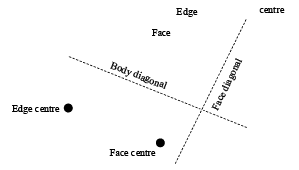1. Total number of edges in a cube = 12
2. Total number of faces in a cube = 6
3. Total number of corners in a cube = 8
4. Total number of body centre in a cube = 1
5. Total number of edge centre in a cube = 12
6. Total number of face centre in a cube = 6
7. Total number of face diagonals in a cube = 6 × 2 = 12
8. Total number of body diagonals in a cube = 4

Types of Lattice and Types of Unit Cell

(i) Primitive or simple Unit Cells: In a primitive unit cell, the same type of particles are present at the all the corners of the unit cell.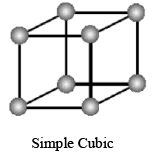(ii) Non-primitive or cantered unit cells: There are three types of non-primitive unit cells as follows:

(a) Face centred: When atoms are present in all 8-corners and six face centres in a cubic unit cell then this arrangement is known as FCC.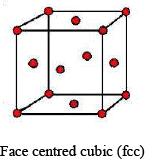(b) End-Centred: When in addition to particles at the corners, there are particles at the centres of the end faces.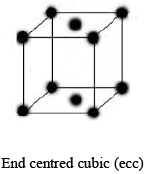(c) Body Centred: When atoms are present at 8 corners as well as in the body centre in a cubic unit cell then this arrangement is known as BCC.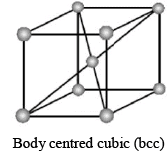Seven Crystal Systems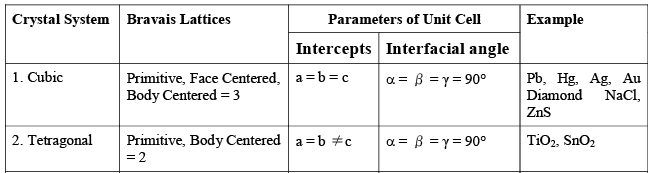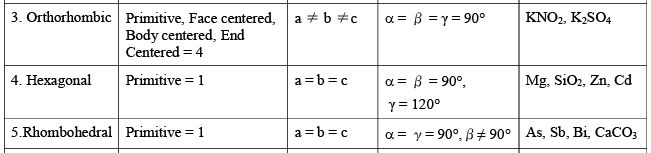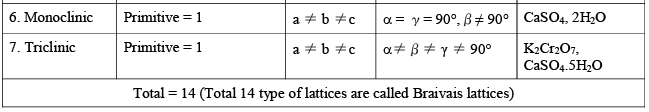Offer running on EduRev: Apply code STAYHOME200 to get INR 200 off on our premium plan EduRev Infinity!

,

,

,

,

,

,

,

,

,

,

,

,

,

,

,

,

,

,

,

,

,

,

,

,

;